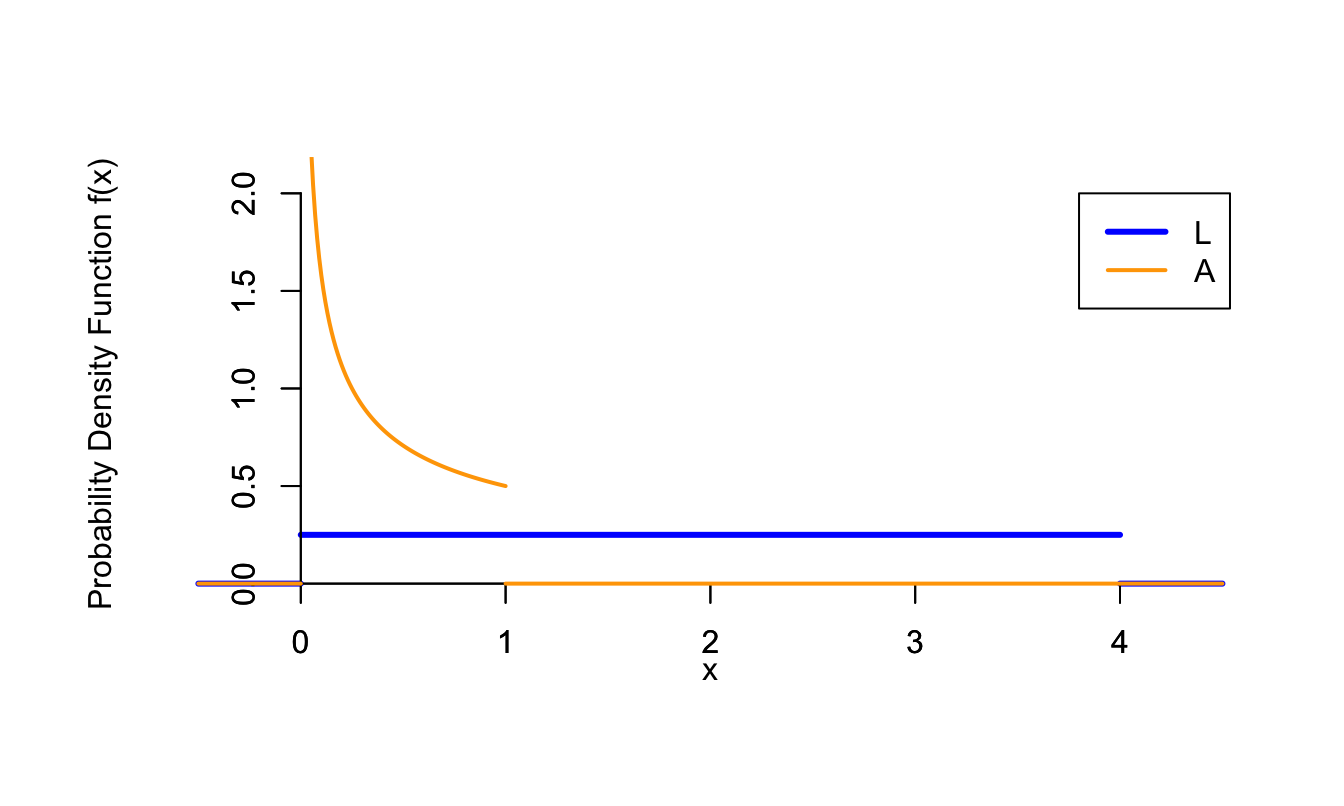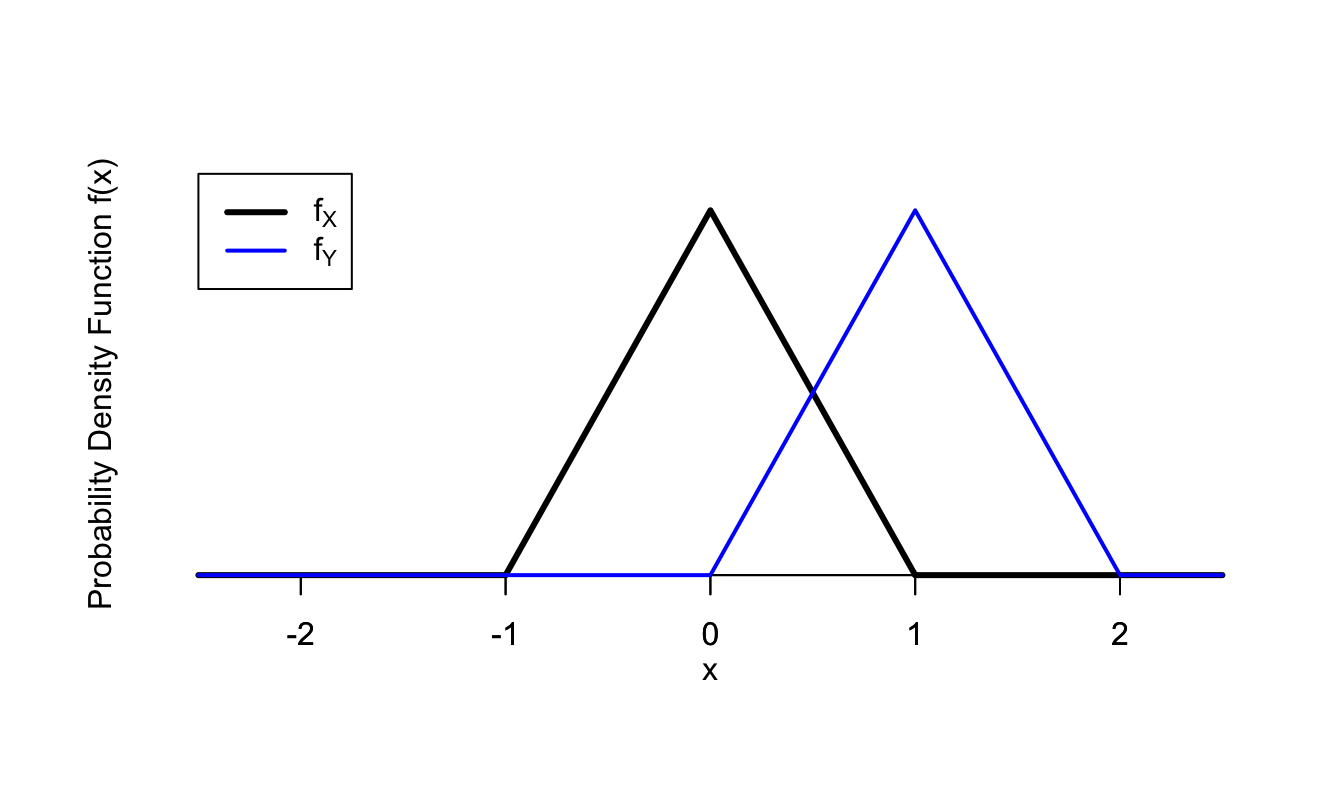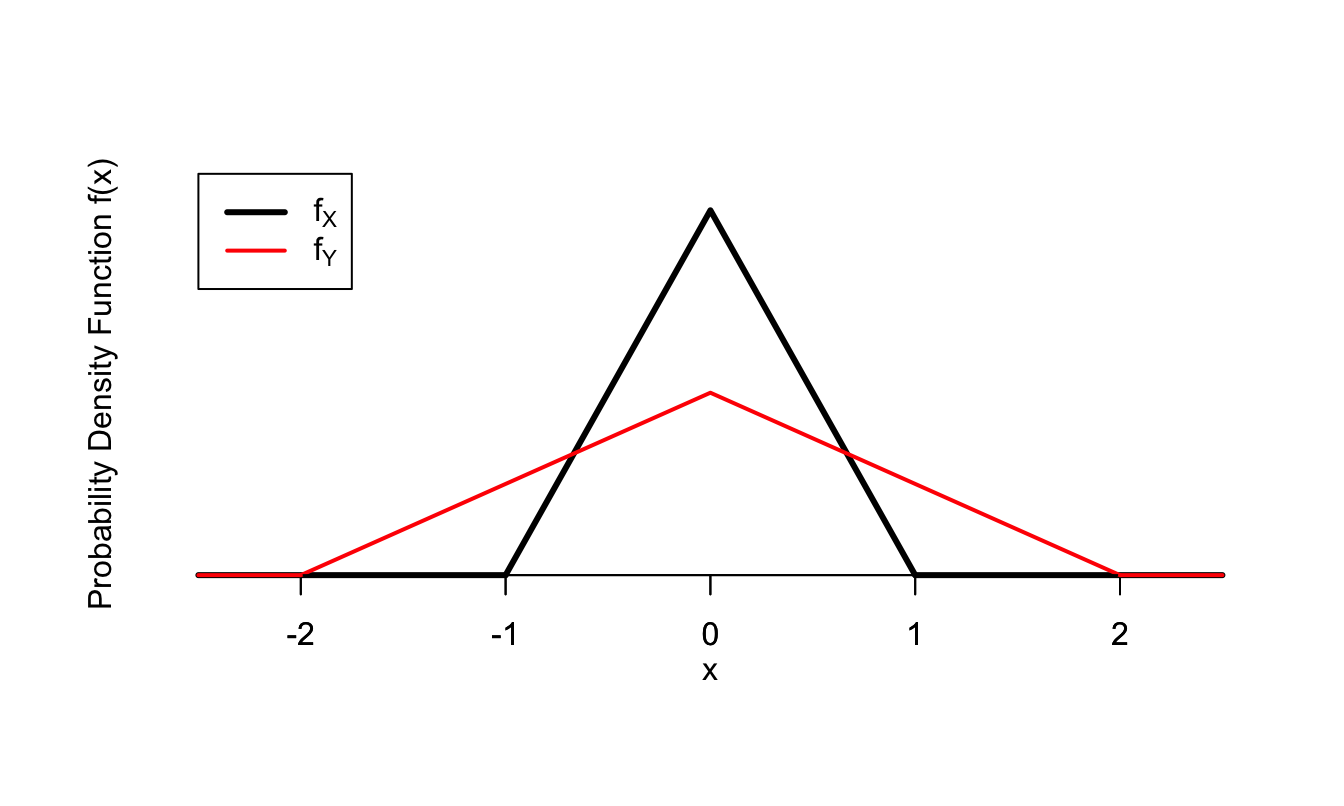# Lesson 36 Transformations

## Motivating Example

You buy fencing for a square enclosure. The length of fencing that you buy is uniformly distributed between 0 and 4 meters. What is the distribution of the area of the enclosure?

Most people realize that the area must be between 0 and 1 square meters, since the length of each side must be between 0 and 1 meters, and the square of a number between 0 and 1 is also between 0 and 1. But how likely are the values in between?

## Theory

We can formalize the above example as follows. The length of fencing, $$L$$, is a $$\text{Uniform}(a=0, b=4)$$ random variable, and we are interested in the distribution of $$A = (L/4)^2$$.

First, let’s simulate the distribution of $$A$$. The Colab below begins with a uniform random variable $$L$$, defines $$A = (L / 4)^2$$, and displays 10000 simulations of both $$L$$ and $$A$$.

In the simulation above, $$L$$ is equally likely to take on all values between 0 and 4. This is not surprising because $$L$$ was defined to be this way (a uniform distribution between 0 and 4). The distribution of $$A$$ is more interesting. It is much more concentrated toward the lower values than the higher values. In hindsight, this makes sense because $$(L / 4)$$ is a number between 0 and 1, and numbers between 0 and 1 get smaller when we square them.

How do we derive the p.d.f. of $$A$$? This is a special case of a more general problem. We have a random variable $$X$$ whose distribution we know, and we want to find the distribution of $$Y = g(X)$$, a transformation of $$X$$. The strategy for these problems is this:

1. Calculate the c.d.f. of $$Y$$ (by rewriting the event in terms of $$X$$ and using the known distribution of $$X$$).
2. Take the derivative to obtain the p.d.f. of $$Y$$ (by Definition 33.2).

Example 36.1 Let’s apply this strategy to the example at the beginning of the lesson.

First, we find the c.d.f. of $$A$$, the area of the enclosure. We write out the definition of the c.d.f., express $$A$$ in terms of $$L$$, and rearrange the expression to make the probability easy to calculate. \begin{align*} F_A(x) &= P(A \leq x) \\ &= P\left((L/4)^2 \leq x\right) \\ &= P(L \leq 4 \sqrt{x}) & \text{(if x \geq 0)} \\ &= \begin{cases} 0 & x < 0 \\ \sqrt{x} & 0 \leq x \leq 1 \\ 1 & x > 1 \end{cases}. \end{align*}

Now we take the derivative to get the p.d.f. $f_A(x) = \frac{d}{dx} F_A(x) = \begin{cases} \frac{1}{2\sqrt{x}} & 0 \leq x \leq 1 \\ 0 & \text{otherwise} \end{cases}.$

We graph the p.d.f.s of $$L$$ and $$A$$ below. Compare these with our simulations above.Figure 36.1: Distributions of $$L$$ and $$A$$

Now, we derive formulas for the two most common types of transformations: shifting by a constant and scaling by a constant.

Theorem 36.1 (Shift Transformation) Let $$X$$ be a continuous random variable with p.d.f. $$f_X$$. The p.d.f. of $$Y = X + b$$ is $\begin{equation} f_Y(y) = f_X(y - b). \tag{36.1} \end{equation}$

Proof. We can express the c.d.f. of $$Y$$ in terms of the c.d.f. of $$X$$: \begin{align*} F_Y(y) &= P(Y \leq y) \\ &= P(X + b \leq y) \\ &= P(X \leq y - b) \\ &= F_X(y - b). \end{align*}

Taking the derivative, we see that the p.d.f. is $f_Y(y) = \frac{d}{dy} F_Y(y) = f_X(y - b).$

The figure below illustrates a hypothetical p.d.f. for a random variable $$X$$ and the corresponding p.d.f. for the shifted random variable $$Y = X + 1$$. Notice how the p.d.f. is exactly the same, except shifted to the right by 1, as we would expect.Figure 36.2: Example of a Shift Transformation

Theorem 36.2 (Scale Transformation) Let $$X$$ be a continuous random variable with p.d.f. $$f_X$$. The p.d.f. of $$Y = aX$$, where $$a > 0$$ is a constant, is $\begin{equation} f_Y(y) = \frac{1}{a} f_X(\frac{y}{a}). \tag{36.2} \end{equation}$

Proof. We can express the c.d.f. of $$Y$$ in terms of the c.d.f. of $$X$$: \begin{align*} F_Y(y) &= P(Y \leq y) \\ &= P(aX \leq y) \\ &= P(X \leq \frac{y}{a}) \\ &= F_X(\frac{y}{a}). \end{align*}

Taking the derivative (don’t forget the chain rule!), we see that the p.d.f. is $f_Y(y) = \frac{d}{dy} F_Y(y) = \frac{1}{a} f_X(\frac{y}{a}).$

The figure below illustrates a hypothetical p.d.f. for a random variable $$X$$ and the corresponding p.d.f. for the scaled random variable $$Y = 2X$$. Notice that the p.d.f. is the same shape, just wider and shorter.Figure 36.3: Example of a Scale Transformation

The factor of $$\frac{1}{a}$$ on the inside in (36.2) is what makes the p.d.f. wider, and the factor of $$\frac{1}{a}$$ on the outside is what makes it shorter. It is necessary to make the p.d.f. shorter if we make it wider, since the total area under a p.d.f. must always be 1.

## Essential Practice

1. You inflate a spherical balloon in a single breath. If the volume of air you exhale in a single breath (in cubic inches) is $$\text{Uniform}(a=36\pi, b=288\pi)$$ random variable, what is the p.d.f. of the radius of the balloon (in inches)?

2. The lifetime of a lightbulb (in hours) follows an $$\text{Exponential}(\lambda=\frac{1}{1200})$$ distribution. Find the p.d.f. of the lifetime of the lightbulb in days (assuming the lightbulb is on 24 hours per day).

3. A lighthouse on a shore is shining light toward the ocean at a random angle $$U$$ (measured in radians), where $$U$$ follows a $$\text{Uniform}(a=-\frac{\pi}{2}, b=\frac{\pi}{2})$$ distribution.

Consider a line which is parallel to the shore and 1 mile away from the shore, as illustrated below. An angle of 0 would mean the ray of light is perpendicular to the shore, while an angle of $$\pi/2$$ would mean the ray is along the shore, shining to the right from the perspective of the figure.Let $$X$$ be the point that the light hits on the line, where the line’s origin is the point on the line that is closest to the lighthouse. Find the p.d.f. of $$X$$.

(Hint: Use trigonometry to write $$X$$ as a function of $$U$$.)

1. The radius of a circle (in inches) is chosen from an $$\text{Exponential}(\lambda=1.5)$$ distribution. Find the p.d.f. of the area of the circle.